Courses

# Post Correspondence Problem and Linear Bounded Automata Computer Science Engineering (CSE) Notes | EduRev

## Computer Science Engineering (CSE) : Post Correspondence Problem and Linear Bounded Automata Computer Science Engineering (CSE) Notes | EduRev

The document Post Correspondence Problem and Linear Bounded Automata Computer Science Engineering (CSE) Notes | EduRev is a part of the Computer Science Engineering (CSE) Course Theory of Computation.
All you need of Computer Science Engineering (CSE) at this link: Computer Science Engineering (CSE)

Part X. Post Correspondence Problem

13 Post Correspondence Problem
Post correspondence problem (PCP) is a problem formulated by E. Post in 1940.
Let us illustrate this problem by an example:

Example 1:
Let there be two series of strings, say x series and y series as shown below: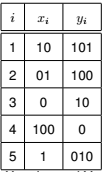Both X series and Y series contains 5 strings.
Let us call the strings in X series as X substrings, and
the strings in Y series as Y substrings.
If we concatenate X substrings x1x5x2x3x4x4x3x4, we get 1010101001000100.
If we concatenate Y substrings y1y5y2y3y4y4y3y4, we get 1010101001000100.
Let us call these strings as x string and y string.
It can be seen that x string and y string are same.
Here we say that this instance of PCP has a solution in the form 15234434.

If we take 23,
x string is, x2x3 =010
y string is, y2y3 =10010.
Here x string and ys tring are different. Hence 23 is not a solution to this instance of PCP.

Example 2:
Find the solution to the instance of PCP given in the following table.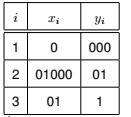Solution,
x2x1x1x3= 010000001
y2y1y1y3= 010000001
Hence, 2113 is a solution to this instance of PCP.

Example 3:
Find why the instance of PCP given below cannot have a solution.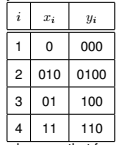It can be seen that for every pair, |xi| > |yi|.
So, in whatever way we concatenate the string, the length of the x string will be longer than the corresponding y string. Thsu there is no solution for this instance of PCP.

Definition of PCP
Let there be two series, x series and y series of size n with same charactere set ∑ with their ith element as xi and yi,respectively; does there exist a solution tha tforms the same x series and y series?
The generic solution of PCP can be written as,
xi1 xi2 xi3........xik = yi1yi2yi3.......yik

Post Correspondence Problem is unsolvable.
This means it is a non computable function. No turing machine exists for PCP.
The proof of this is beyond the scope of our study.

Use of PCP
Other problems can be reduced to PCP and we can declare them as unsolvable or undecidable.

Part XI. Linear Bounded Automata

Consider the following figure: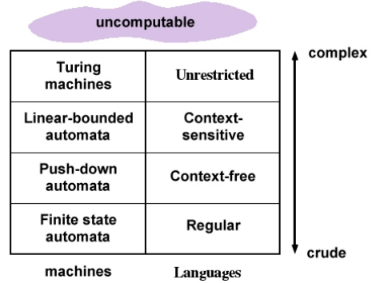We learned in Chomsky classification that context sensitive languages (Type-1) are produced from context sensitive grammars.
Context sensitive languages are recognised using linear bounded automata.

The following is an example for a Context Sensitive grammar:
S → aBCT|aBC
T → ABCT|ABC
BA → AB
CA → AC
CB → BC
aA → aa
aB → ab
bB → bb
bC → bc
cC → cc
in this grammar, LHS of a production is not longer than the RHS.
The language, L = {anbncn|n > 0} is a context sensitive language.

14 Linear Bounded Automata (LBA)
Context sensitive languages are recognised using linear bounded automata (LBA).
Here input tape is restricted in size. A linear function is used for restricting the length of the input tape.
Many compiler languages lie between context sensitive and context free languages.
A linear bounded automation is a non deterministic Turing machine which has a single tape whose length is not infinite
but bounded by a linear function.

Formal Definition
A linear bounded automation is defined as,
M = (Q,∑, Γ, δ, q0, #, <,>,F),
where
Q is a set of states,
∑ is a set of input symbols,
Γ is a set of tape symbols, including #,
δ is the set of transition functions from
(Q × Γ) → (Q × Γ × {L, R, N}),
q0 is the start state,
# is the blank symbol,
< is the left end marker in the input tape,
> is the right end marker in the input tape,
F is the final state.
Following diagram shows a linear bounded automation,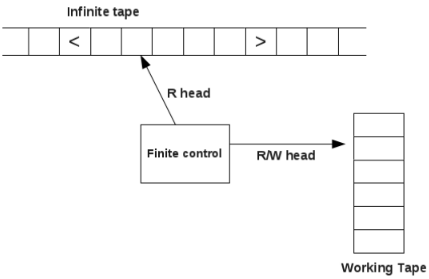When an input string is processed using an LBA, input string is enclosed between < and > end markers. The end marker < prevents the R head from getting off the left end of the tape. The right end marker > prevents the R head from getting off the right end of the tape. On the input tape, head does not write. Also on the input tape, head does not move left. All the computation is to be done between end markers < and >. On the working tape head can read and write without any restriction.
Thus LBA is same as a Turing machine except that head can move only within the end markers.

Example:
Consider an LBA defined as,
Q= {q0, q1, q2, q3, q4}
∑= {a, b, c}
Γ= {a, b, c, #}
q= s
δ(q0, [) = (q1, [, R)      δ(q1, ]) = (q1, ],Y )     δ(q1, #) = (q1, #, R)
δ(q1, a) = (q2, #, R)    δ(q2, a) = (q2, a, R)   δ(q2, #) = (q2, #, R)
δ(q2, b) = (q3, #, R)    δ(q3, b) = (q3, b, R)   δ(q3, #) = (q3, #, R)
δ(q3, c) = (q4, #, L)     δ(q4, c) = (q4, c, L)   δ(q4, b) = (q4, b, L)
δ(q4, a) = (q4, a, L)    δ(q4, #) = (q4, #, L)   δ(q4, [) = (q1, [, R)
In the above, the transition δ(q1, a) = (q2, #, R) means,
LBA on state q3, head points to symbol b, remains in state q3, replaces b with b and head turns towards right by one cell.

Offer running on EduRev: Apply code STAYHOME200 to get INR 200 off on our premium plan EduRev Infinity!

## Theory of Computation

18 videos|44 docs|39 tests

,

,

,

,

,

,

,

,

,

,

,

,

,

,

,

,

,

,

,

,

,

;